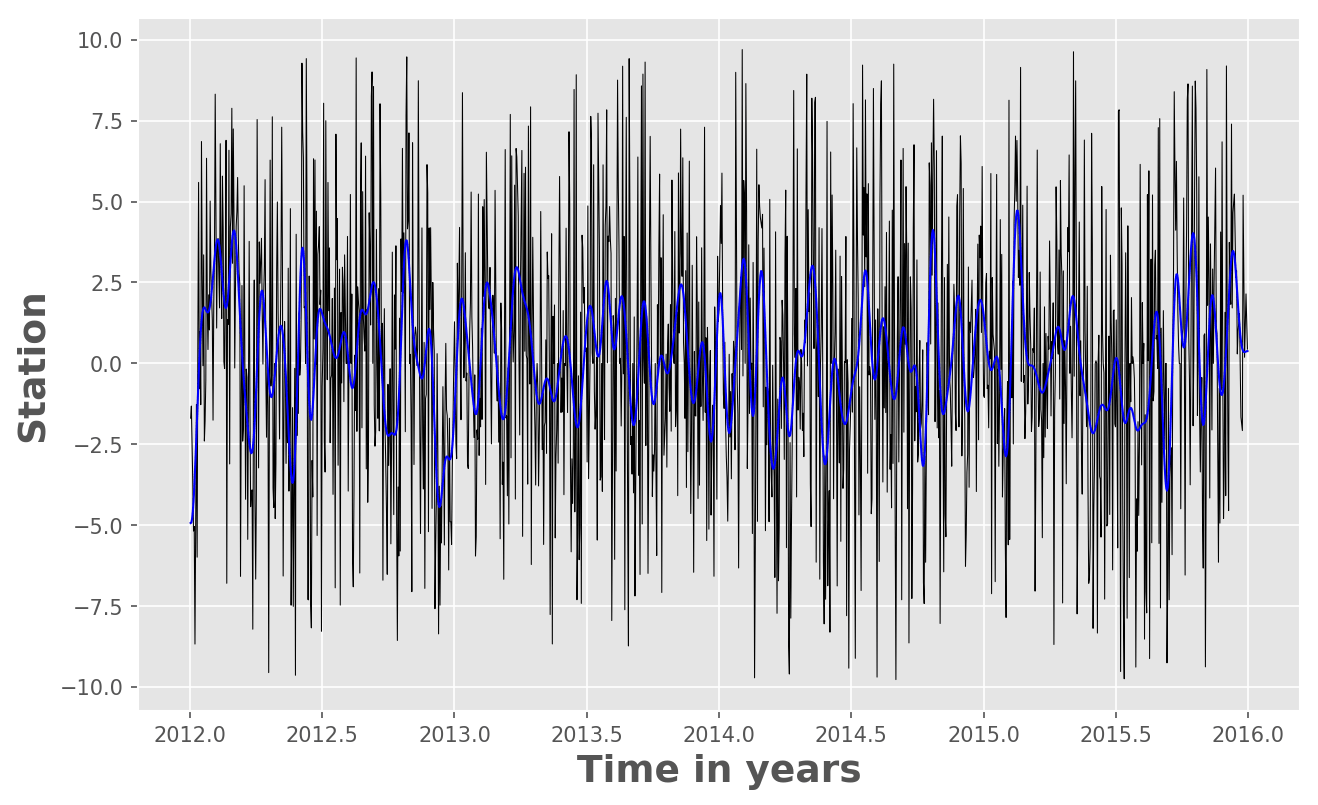# Time Series Analysis in Python: Filtering or Smoothing Data (codes included)

In this post, we will see how we can use Python to low-pass filter the 10 year long daily fluctuations of GPS time series. We need to use the “Scipy” package of Python.

In this post, we will see how we can use Python to low-pass filter the 10 year long daily fluctuations of GPS time series. We need to use the “Scipy” package of Python.

## Similar posts

#### Convert any text to lifelike speech using amazon polly

The only important thing to keep in mind is the understanding of Nyquist frequency. The Nyquist or folding frequency half of the sampling rate of the discrete signal. To understand the concept of Nyquist frequency and aliasing, the reader is advised to visit this post. For filtering the time-series, we use the fraction of Nyquist frequency (cut-off frequency).

## Code Description

Following are the codes and line by line explanation for performing the filtering in a few steps:

### Import Libraries

• import `numpy` module for efficiently executing numerical operations
• import the `pyplot` from the matplotlib library
• predefine figure window size, and default figure settings
• use matplotlib `ggplot` style. I personally like to use “ggplot” style of graph for my work but it depends on the user’s preference whether they wanna use it.
``````import numpy as np
import matplotlib.pyplot as plt
from scipy import signal

from matplotlib import rcParams
rcParams['figure.figsize'] = (10.0, 6.0) #predefine the size of the figure window
rcParams.update({'font.size': 14}) # setting the default fontsize for the figure
rcParams['axes.labelweight'] = 'bold' #Bold font style for axes labels
from matplotlib import style
style.use('ggplot')
``````

We load the data in the mat format (skipped) but this code will work for any sort of time series. If you have MiniSeed data, you can easily convert that to the MATLAB or mat format using the following utility:

``````# loading data part skipped (can be done using scipy for mat format data)
dN=np.array(data['dN'])
dE=np.array(data['dE'])
dU=np.array(data['dU'])
slat=np.array(data['slat'])
slon=np.array(data['slon'])
tdata=np.array(data['tdata'])
stn_name=np.array(stn_info['stn_name'])
stns=[stn_name[i] for i in range(len(stn_name))]
``````

### Visualizing the original and the Filtered Time Series

``````fig = plt.figure()
indx=np.where( (tdata > 2012) & (tdata < 2016) )
ax.plot(tdata[indx],dU[indx,0],'k-',lw=0.5)
``````

### Filtering of the time series

``````fs=1/24/3600 #1 day in Hz (sampling frequency)

nyquist = fs / 2 # 0.5 times the sampling frequency
cutoff=0.1 # fraction of nyquist frequency, here  it is 5 days
print('cutoff= ',1/cutoff*nyquist*24*3600,' days') #cutoff=  4.999999999999999  days
b, a = signal.butter(5, cutoff, btype='lowpass') #low pass filter

dUfilt = signal.filtfilt(b, a, dU[:,0])
dUfilt=np.array(dUfilt)
dUfilt=dUfilt.transpose()
``````
• Continue plotting on the exisitng figure window
``````ax.plot(tdata[indx],dUfilt[indx],'b',linewidth=1)

ax.set_xlabel('Time in years',fontsize=18)
ax.set_ylabel('Stations',fontsize=18)
plt.savefig('test.png',dpi=150,bbox_inches='tight')
``````

## Output Figure:Tags:

Categories:

Created on:#### Disclaimer of liability

The information provided by the Earth Inversion is made available for educational purposes only.

Whilst we endeavor to keep the information up-to-date and correct. Earth Inversion makes no representations or warranties of any kind, express or implied about the completeness, accuracy, reliability, suitability or availability with respect to the website or the information, products, services or related graphics content on the website for any purpose.

UNDER NO CIRCUMSTANCE SHALL WE HAVE ANY LIABILITY TO YOU FOR ANY LOSS OR DAMAGE OF ANY KIND INCURRED AS A RESULT OF THE USE OF THE SITE OR RELIANCE ON ANY INFORMATION PROVIDED ON THE SITE. ANY RELIANCE YOU PLACED ON SUCH MATERIAL IS THEREFORE STRICTLY AT YOUR OWN RISK.